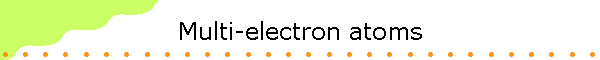The Schroedinger equation for one electron atoms like hydrogen can be solved using the technique of separation of variables.  To deal with a two electron atom like helium, we have to take into account not only the attraction between the electrons and the nucleus, but also the repulsion between electrons.  This makes the Schroedinger equation more difficult to solve.  One has to either use approximation techniques or numerical techniques.

However, some properties of all atoms can be understood in terms of the general structure of the solution of the Schroedinger equation for the hydrogen atom, rather than from detailed calculations.  We can learn enough from these general properties to begin to see why atoms behave as they do in chemical reactions.

To study multi electron atoms, imagine that we start with hydrogen and add electrons one at a time.  At the same time we increase the number of protons and neutrons in the nucleus to keep the atom electrically neutral and the nucleus stable.  We will assume that as we add each electron, it falls down to the lowest energy eigenstate available.  If we start with a nucleus with one proton, and drop in one electron, the electron eventually falls down to the n = 1 state.   Its energy is  E1 = -13.6 eV.  As we add a proton (and two neutrons) to form a helium nucleus and drop in another electron, we can expect the electron to also fall down to the n = 1 state.  The extra Coulomb attractive force of the two protons in the nucleus strengthens the binding of the electrons, but the repulsive force between the two electrons weakens it.  Experimentally, it takes 24.6 eV to remove an electron from helium, while only 13.6 eV are needed for hydrogen.  Thus the electrons are more tightly bound in helium, and we see that the extra Coulomb attraction to the nucleus is more important than the repulsion between electrons.

Using helium as a guide, we should expect that when we go to lithium with 3 protons in the nucleus, the increased Coulomb attraction to the nucleus should cause lithium’s three electrons to be even more tightly bound than helium’s two.  This would lead us to predict that it takes even more than 24.6 eV to pull one of the electrons out of lithium.

This is not a good prediction.  Experimentally, the amounts of energy needed to remove electrons from lithium one at a time are 5.39 eV, 75.26 eV and 121.8 eV.  While two of lithium’s electrons are tightly bound, one is very loosely bound, requiring less than half the energy to remove than it takes to remove the electron from hydrogen.  A possible explanation for the loose binding of lithium’s third electron is that, for some reason, the electron does not fall down to the lowest energy n = 1 state.  It appears to be hung up in one of the higher energy, less tightly bound n = 2 states.  For n = 2 there are 4 different sets of quantum numbers and 4 different wave functions.

Pauli Exclusion Principle

But why would the third lithium electron not fall down to the low energy n = 1 state?  In 1925, two separate ideas provided the explanation.  Wolfgang Pauli proposed that no two electrons were allowed to be in exactly the same state.  This is known as the Pauli exclusion principle.  But the exclusion principle seems to go too far, because in helium, both electrons are in the same n = 1, l = 0, m = 0 state.  If you cannot have two electrons in exactly the same state in an atom, then something must be different about the two electrons in helium.

Electron Spin

To explain this difference between the two electrons, two graduate students, Samuel Goudsmit and George Uhlenbeck, proposed that the electron had its own internal angular momentum.  This became known as spin angular momentum.  The special feature of the electron’s spin is that it has two allowed projections along any axis, which we call spin up and spin down.  In helium you could have two electrons in the same n = 1 state if they had different spin projections, because then they would not be in identical states.  A state would be characterized by 4 quantum numbers, n, l, m, and ms, where ms tells us about the projection of the electron's spin on the z-axis.  Because the electron spin has only two allowed projections along any axis, we cannot add a third electron to the n = 1 state.  Lithium’s third electron must stop at one of the higher energy n = 2 states.  Its energy is much less negative and therefore this electron is much less tightly bound than the first two electrons that went down to the n = 1 state.

The spin of a particle is closely related to its properties in statistical mechanics.  Particles with half-integer spin obey Fermi-Dirac statistics, and are known as fermions.  They are subject to the Pauli exclusion principle, which forbids them from sharing quantum states, and they are described in quantum theory by "antisymmetric states".  Particles with integer spin, on the other hand, obey Bose-Einstein statistics, and are known as bosons.  These particles can share quantum states, and are described using "symmetric states".

 The periodic table As we go to larger atoms, adding electrons one at a time, the n = 2 states begin to fill up.  Since there are four different values for (l,m), each with two allowed spin states, up to 8 electrons can fill the n = 2 states.  All the states with quantum number n = 2 are said to be in the n = 2 shell.  A shell refers to all the states with the same quantum number n.  A subshell refers to all the state with the same quantum numbers n and l.  When the n = 2 states are all filled we get to the element neon with two n = 1 electrons and eight n = 2 electrons.  Neon is an inert noble gas that is chemically similar to helium.  Adding one more electron by going to sodium, the eleventh electron has to go up into the n = 3 energy level since both the n = 1 and n = 2 states are full.  This eleventh electron in sodium is loosely bound like the third electron in lithium, with the result that both lithium and sodium have similar chemical properties.  They are both strongly reactive metals.  The table on the right shows the electron structure and the binding energy of the last (most loosely bound) electron for the first 36 elements in the periodic table. The general features of this table are that the lowest energy levels fill up first, and there is a large drop in binding energy when we start filling a new energy level.  We see these drops when we go from the inert gases helium, neon, and argon to the reactive metals lithium, sodium and potassium.  We can see that this sudden change in binding energy leads to a significant change in the chemical properties of the atom.  A closer look at the table shows that there is a relatively steady uniform increase in the electron binding as the energy level fills up.  The binding energy goes from 5.39 eV for lithium in fairly equal steps up to 21.56 eV for neon as the n = 2 energy level fills.  The pattern is more or less repeated as we go from 5.14 eV for sodium up to 15.76 eV for argon while filling the n = 3 energy level.  It repeats again in going from 4.34 eV for potassium up to the 14.00 eV for krypton. A closer look also uncovers some exceptions to the rule that the lower n-states fill first.  The most notable exception is at potassium, where the n = 4 state with l = 0 begin to fill before the n = 3 states with l = 2.  To understand why the binding energy gradually increases as a shell fills up, and why the n = 3 , l = 2 states fill up late, we have to take a closer look at the electron wave functions and see how their shape affects the binding energy.  To do this it is useful to introduce the concepts of electron screening and effective nuclear charge.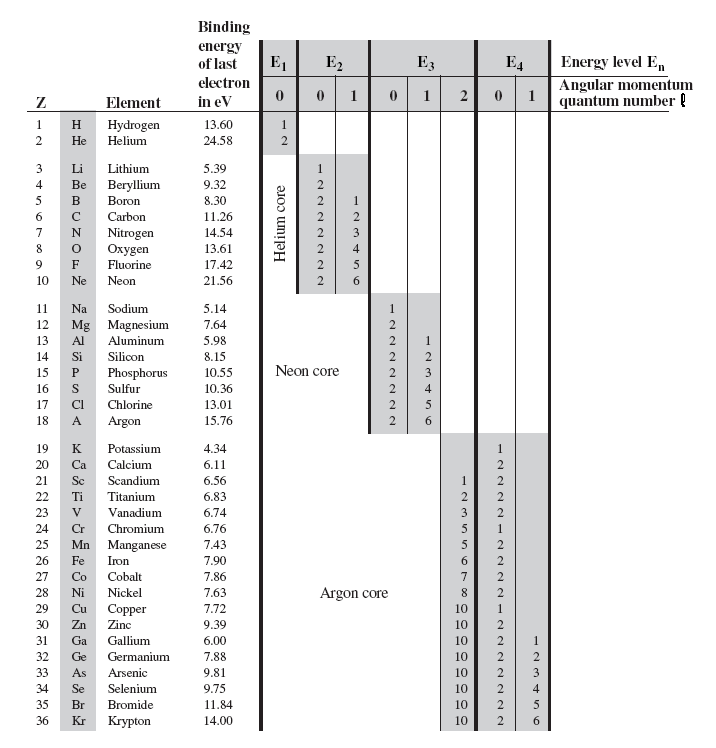Electron Screening

In our discussion of the binding energy of the two electrons in helium, we pointed out that there was a competition between the increased Coulomb attractive force due to the nucleus and the repulsion between the electrons.  We could see that the increased attraction was more important because helium’s two electrons are each more tightly bound to the nucleus than hydrogen’s one electron.  It requires 24.5 eV to remove an electron from helium and only 13.6 eV from hydrogen.  The following argument provides an explanation of this increased binding of helium’s electron.  Since the two electrons have the same n = 1 wave functions, half the time a given electron is closer to the nucleus than its partner and feels the full force of the nuclear charge +2qe.  But half the time it is farther away, and the net charge attracting it toward the nucleus +2qe is reduced by the other electron’s charge –1qe for a total +1qe. Thus, on the average, the electron sees an effective charge of approximately 1.5qe.  This is greater than the charge +1qe seen by the single electron in hydrogen, and thus results in a lower binding energy.  We account for the repulsion of the other electron by saying that the other electron screens the nucleus, reducing the nuclear charge from +2qe to an effective value of approximately 1.5qe.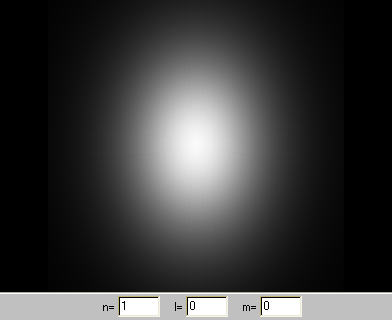Effective Nuclear Charge

The ground state energy of a hydrogenic atom with Z protons in the nucleus is E1 = -Z2*13.6 eV.  The equation En =  -Z2*13.6 eV/n2 suggests that if an electron with principle quantum number n in a multi-electron atom sees an effective nuclear charge Zeff, then the electron's binding energy should be approximately En = -Zeff2*13.6 eV/n2.

Helium

Trying out this idea on helium, where we estimated Zeff to be about 1.5, we get E1 = -(1.5)2* 13.6 eV = -30.6 eV as the estimated binding energy of helium.  This estimate of 30.6 eV is about 25% too high since the experimental value is only 24.6 eV.  We can take this to simply mean that our estimate of Zeff = 1.5 for helium is a bit too crude.  Our simple arguments about screening are not a substitute for an accurate calculation using Schrödinger’s equation.

We can use En = -Zeff2*13.6 eV/n2 to calculate an effective nuclear charge Zeff  for the outermost electron in an element using its measured binding energy.  Doing this for helium yields

-24.58 eV = -Zeff2*13.6 eV,
Zeff = 1.34.

The value of 1.34 is not too far from the guess of 1.5.  The electron screening is a bit more effective than we had predicted.

Lithium

We will now see that various features of the periodic table begin to make sense when viewed in terms of electron screening and the structure of the electron wave functions.  Let us start off with lithium where the last electron is in the n = 2, l = 0 state and has a binding energy of 5.39 eV.  Since this electron is in a n = 2 energy level, our formula for Zeff yields

-5.39 eV = -Zeff2*13.6 eV/4,
Zeff = 1.26,

where we used 13.6/4 eV = 3.40 eV rather than 13.6 eV because we are discussing a n = 2 electron.

In a nucleus with 3 protons, why does the n = 2 electron only see an effective charge of 1.26 qe?  The answer lies in the shape of the n = 1 and n = 2, l = 0 wave functions.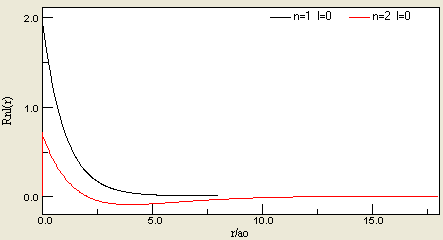The first two electrons in lithium are in the n = 1 state.  Its probability density consists of a small spherical ball centered on the nucleus.  The third electron, the one whose binding energy we are discussing, is in the n = 2,  l = 0 state.  Its probability density consists of a larger spherical ball surrounded by a spherical shell.  The electron in this state spends a considerable amount of the time outside the smaller spherical ball of the two n = 1 electrons.  Thus much of the time the third electron sees only an effective nuclear charge of about +1 qe.  Some of the time, however, the third electron is near the nucleus feeling the full nuclear charge of +3 qe.  It is not difficult to believe that the average nuclear charge seen by the third electron is +1.26 qe.

Beryllium

When we went from one n = 1 electron in hydrogen to two n = 1 electrons in helium, the binding energy about doubled, from 13.6 eV to 24.6 eV.  In going from one n = 2 electron in lithium to two n = 2 electrons in beryllium, the binding energy increases from 5.39 eV to 9.32 eV.  Again the electron binding energy almost doubles as we go from one to two electrons with the same n and l quantum numbers.

 Boron When we go from beryllium to boron, we add a third electron to the n = 2 energy level.  From our experience with beryllium, we could expect another significant increase in binding energy, up to perhaps 13 eV or 14 eV.  But instead the binding energy drops from 9.32 eV down to 8.30 eV.  What causes this drop? At boron, both the n = 2 , l = 0 states are already full and the electron has to go into one of the n = 2 , l = 1 states.  All the electron wave functions with non-zero angular momentum are zero at the origin.  The greater the angular momentum, the farther the electron is kept away from the nucleus.  An electron with an l > 0 wave function will thus be effectively screened by electrons in the l = 0 states, where the electron spends a lot of time close to the nucleus.  Thus we expect that electrons with quantum number n and l > 0 wave functions are less tightly bound than those with l = 0 wave functions.  This shows up with the drop in binding energy in going from beryllium to boron.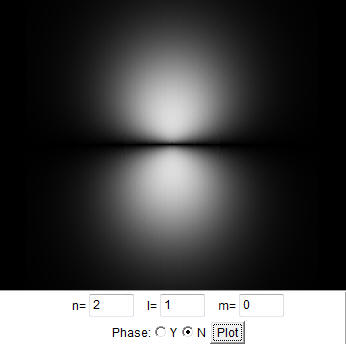Up to Neon

For all atoms beyond helium, there is a core consisting of the nucleus and the two tightly bound n = 1 electrons.  As the charge on the nucleus increases, the size of the n = 1 wave functions shrinks, and they are penetrated less and less by the outer electrons.  We can think of this helium core as acting as the effective nucleus for the larger atoms.  As we go from boron to neon, the charge on the helium core increases from 3 qe to 8 qe as the n = 2 , l = 1 state fill up.  This increase in the charge of the core causes a more or less gradual increase in the binding energy, from 8.30 eV up to 21.56 eV.  The one exception is the slight drop in binding energy as we go from nitrogen to oxygen.  The arguments we have made so far are not detailed enough to explain this drop.

Sodium to Argon

We get the expected large drop in binding energy as we go from neon to sodium and start filling the n = 3 states.  The n = 3 , l = 0 states are full at magnesium and we get a small drop in binding energy as the non-zero angular momentum states n = 3, l = 1 start to fill at aluminum.  Again the angular momentum keeps the electrons away from the nucleus and increases the screening.  As the n = 2,  l = 1 states fill up, they are building a structure on the ever shrinking neon core.  The charge on the neon core increases from 3 qe at aluminum to 8 qe at argon, again causing a gradual buildup of the electron binding energy from 5.98 eV to 15.76 eV.  There is even the slight glitch going from phosphorous to sulfur that mirrors the glitch from nitrogen to oxygen.

Potassium to Krypton

The first major break in the pattern of filling the lower energy levels occurs at potassium.  At potassium the n = 3, l = 2 levels remain unfilled while the last electron goes into the n = 4, l = 0 state.  At this point the screening due to angular momentum has become more important than the principle quantum number n.  The l = 2 wave functions have a large node at the nucleus, and a l = 2 electron cannot get near the nucleus to feel the now large nuclear charge.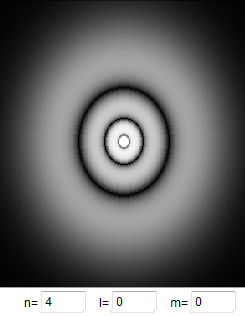Even though an n = 4 , l = 0 electron has a large principal quantum number, its wave function has a non zero value at the nucleus.  Some of the time this electron feels the full charge of 19 qe for potassium, and this increases the binding energy beyond that of the n = 3, l = 2 wave function.  At calcium, the n = 4, l = 0 state is full, and now the five n = 3, l = 2, m = +2, +1, 0, -1, -2 states begin to fill up.  There is room for 10 electrons in these 5 states, and that takes us down to zinc.  As the n = 3, l = 2 states fill up underneath the n = 4, l = 0 states, there is little change in the outer electron structure and the binding energy increases slowly.  The result is that the 10 elements from scandium to zinc have similar chemical properties, all are metals.  In some periodic tables, these elements are shown as the first set of transition elements.  As we go from gallium to krypton we have the familiar pattern of the n = 4, l = 1 states filling up.  There is a gradual increase in binding energy from 6.00 eV at gallium to 14.00 eV at the noble gas krypton.  There is even the slight glitch in binding energy going from arsenic to selenium that mirrors the glitches from phosphorus to sulfur, and from nitrogen to oxygen.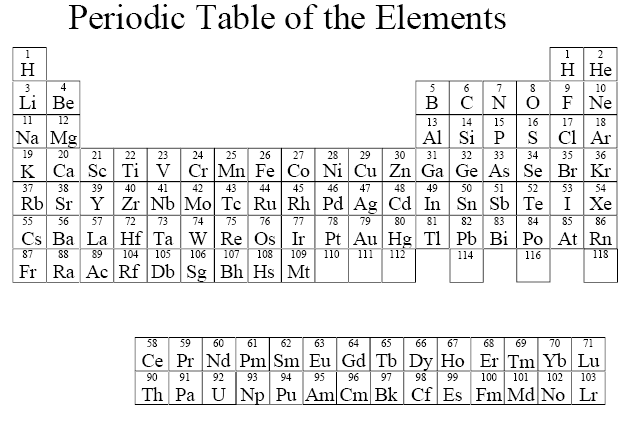Summary

At this point it should be clear that the structure of the periodic table of the elements arises from the allowed electron wave functions.  Because of the exclusion principle, no two electrons can be in the same state.  But because electron spin has two allowed states, up to two electrons can fit into each wave pattern.  In general, as we go to atoms with more electrons, the lowest energy patterns fill up first, and there is a significant change in chemical properties when a new energy level begins to fill.  But the angular momentum of the wave function also plays a significant role. The l = 0 function can penetrate down to the nucleus, where the electron feels the full strength of the nuclear charge. The l > 0 functions have a node at the nucleus, and the full nuclear charge is screened by l = 0 electrons.  The effect of angular momentum shows up most noticeably at potassium and calcium, where the two n = 4, l = 0 states fill before the n = 3, l = 2 states.  Because of the extra angular momentum of the l = 2 electrons, the l = 2 wave functions have an extra large node at the nucleus, keeping these electrons farther away and more effectively screened.
As we get to the heavier elements in the periodic table, those beyond krypton, the energy levels get closer together and the binding energy depends more on the detailed structure of the wave functions.  As a result, it becomes more difficult to predict how the states will be filled and to estimate what the binding energies should be.  But despite this, we have been able to go a long way in explaining the structure of the periodic table from a few simple arguments about the shape of the electron wave function in hydrogen, and the idea of electron screening.

Notation:

An electron configuration is an enumeration of the values of n and l for all electrons of an atom.  The standard notation to describe atomic electron configurations is to denote each subshell by a number equal to its the principal quantum number n, a letter referring to its angular momentum quantum number l and a superscript giving the number of electrons in the subshell.

Examples:

 Element # of Electrons in Element Electron Configuration He 2 1s2 Li 3 1s22s1 Be 4 1s22s2 O 8 1s22s22p4 Cl 17 1s22s22p63s23p5 K 19 1s22s22p63s23p64s1

The electron subshells of atoms in their ground state are filled one electron at a time by putting each electron into the state with the lowest available energy.  The energy ordering of the subshells can be remembered from the diagram below.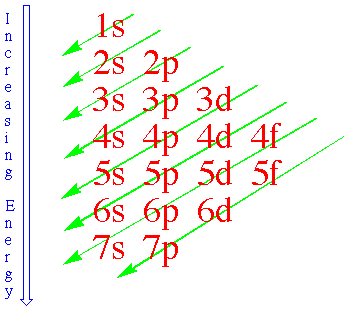Electrons in a subshell with l = 0 have wave functions and probability densities that are spherically symmetric.  The probability density of an electron in a subshell with l > 0 varies with angle.  However, when a subshell is completely filled, then the probability of finding an electron a given distance from the nucleus is the same for any angle.

Electrons are point particles.  We either find an electron at a given position, or we do not find it.  If we find an electron, it has charge -qe.  Only if we average the amount of charge found in a given volume V over time can we define a charge density or charge distribution Q/V.  This average charge density is proportional to the probability density.  For a closed subshell the electron charge distribution is spherically symmetric.

Ionic Bonding

Suppose we place a sodium atom next to a chlorine atom.  What will happen?  The sodium atom has one loosely bound electron in the n = 3, l = 0 state.  The binding energy of this electron is 5.14 eV.  The chlorine atom has seven n = 2 electrons all tightly bound because of the increase in the effective nuclear charge seen by these electrons.  It requires 13.01 eV to remove an electron from chlorine.  If the sodium and the chlorine atom are brought close enough together, the loosely bound outer sodium electron can lose energy by moving into the remaining n = 2  state in the chlorine atom.  We end up with a negative chlorine ion Cl-, where all the n = 2 states are full, and a positively charged sodium ion Na+ ion which has lost its outer electron.  These charged ions then attract each other electrically to form the sodium chloride molecule NaCl, which is common table salt.  Sodium chloride is a typical example of ionic bonding.  The class of elements like lithium, sodium, magnesium, aluminum, etc. that have one, two, or even three loosely bound electrons, tend to give up these electrons in a chemical reaction.  These are called metals.  Those elements like oxygen, fluorine and chlorine, which have nearly full shells and tightly bound electrons, tend to take up electrons in a chemical reaction and are called non metals.  When metals and non metals combine, held together by ionic bonding, you get a compound called a salt.

Problem:

How many subshells and electron states are in the n = 5 shell?

• Solution:

Each subshell is characterized by a value of n and l.  For n = 5 there are 5 possible values of l.  We have 5 subshells.
l = 0: m = 0, ms  =1/2, -1/2.  There are 2 possible electron states.
l = 1: 3 m values are possible, 2 ms values are possible.  There are 3*2 = 6 possible states.
l = 2: 5 m values are possible, 2 ms values are possible.  There are 5*2 = 10 possible states
l = 3: 7 m values are possible, 2 ms values are possible.  There are 7*2 = 14 possible states
l = 4: 9 m values are possible, 2 ms values are possible.  There are 9*2 = 18 possible states
Total number of possible states: 2 + 6 + 10 +14 + 18 = 50.